Keyah Math Module 5, Level 2

Mathematical Content : Circumference of a circle, geometry, volume of a sphere

Objective: Use the method of Eratosthenes to make an estimate of the circumference of the Earth using locations in Arizona; use this estimate to compute its radius and volume

#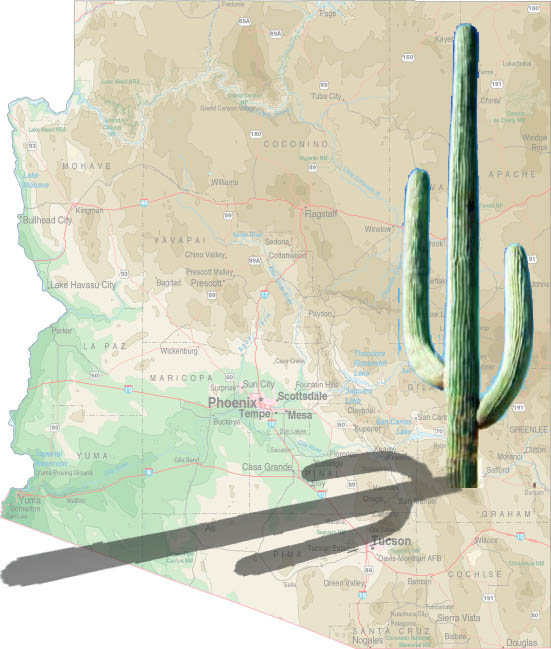Introduction

This set of exercises gives you the opportunity to use the method of Eratosthenes locally (Arizona) to estimate the circumference of the Earth.  Around 250 BC, at noon on the day of the summer solstice (when the sun is at its highest point in the Northern Hemisphere) in Syrene, Egypt, sunlight filled the vertical shaft of a well; this indicates that the sun is directly overhead, so a vertical pole would cast no shadow. Eratosthenes, who lived in Alexandria, heard of this from a traveler. So on the same day, different year, he noticed that in Alexandria, some 800 kilometers (km) away, a vertical pole cast a shadow. From these observations, he deduced that the earth is curved and he estimated the circumference:  Module KM#4 provides a detailed description of this. (You can refer to that module for hints.) This module is slightly different in that the locations we use are farther north than the ones Eratosthenes used, so the angles are different. We suppose the measurements here are taken at noon on the fall equinox, this is when the sun is directly above the equator on its path from summer to winter in the Northern Hemisphere. We use stations just south of Kaibito, Arizona  and in Superior,Arizona  (instead of Syrene and Alexandria, in Egypt).  Kaibito is a small village on the Navajo Reservation in northern Arizona, almost due north of Superior, this will be one of the stations we use to complete these calculations. The other station is Superior, Arizona, a copper mining town about 60 miles east of Phoenix.

Using Math to Estimate the Circumference, Radius and Volume of the Earth

Vertical poles are erected at both stations and angles are measured similar to the way they were measured in by Eratosthenes. In the (not to scale) Figure 1: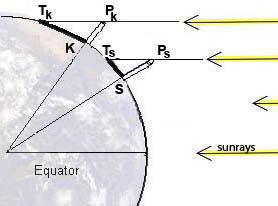S = the base of the pole in Superior, AZ;

K = the base of the pole in Kaibito, AZ;

TS = the tip of the shadow cast by the pole in Superior;

TK = the tip of the pole's shadow in Kaibito;

PS = the top of the pole in Superior;

PK = the top of the pole in Kaibito;

E = the center of the Earth

The measurements give these values: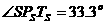(try to measure this yourself on the next equinox);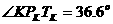The direct distance from Superior to Kaibito is 367 km.

 Question 1: What is the circumference of the Earth? Hints: First label the figure with the measurements you have been given.  Can you determine the measure of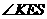?  Now follow the method of Eratosthenes to obtain your own estimation of the circumference of the Earth.
 Question 2: What is the radius of the Earth? A. Compute the radius of the Earth using your estimate of the circumference. (Round your answer to 1 decimal place.)  Circumference of a circle: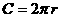where r is the radius. B. Convert your answer to part A to meters (1 km = 1000 meters).
 Question 3: What is the volume of the Earth? Compute the volume of the Earth using your answer to Exercise 2 B. (Round your answer to 3 decimal places.)   Volume of a Sphere: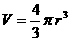where r is the radius.

Here are some follow-up exercises:

 1. If you know some trigonometry you can use the information to determine the radius and then the circumference.  Recall that the arc length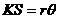where q is the measure ofin radians. Convert degrees to radians and the solve for r.  Now use this to determine the circumference and volume. 2. Look at a topographical map of New Mexico or Arizona and choose two locations to use to measure the circumference of the Earth. You’ll need to know the latitudes and longitudes of your chosen locations in order to find the angles, and the places must be on approximately the same longitude (why?). You can also use the internet to find this information, go to www.topozone.com. Don’t choose places too close together because this will decrease your accuracy. You’ll need to estimate the direct distance between the locations—use the map scale or find it on the internet. 3. There are other variations on this exercise, for example, you can change the time of year, maybe to a solstice. You’ll need the fact that the Earth’s axis is tilted 23.5o to the plane of the solar system. Or you can change the location to your favorite region of the World.

This material is based upon work supported by the National Science Foundation under Grant GEO-0355224. Any opinions, findings, and conclusions or recommendations expressed in this material are those of the authors and do not necessarily reflect the views of the National Science Foundation.